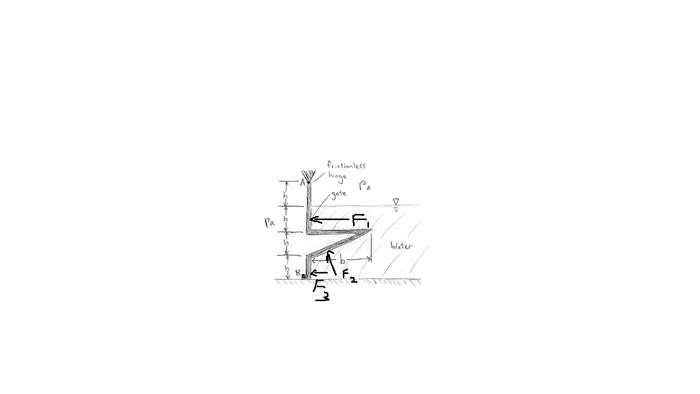# Fluid Statics

eurekamehI've figured out the resultant force on the gate by adding the magnitudes of F1, F2, and F3. However, I'm trying to figure out the distance b such that there is absolutely no force acting on the stop at point B. Is this even possible? It seems like it isn't, since all forces are pointing to the left and there has to always be a reaction force at B.

## Answers and Replies

Homework Helper
Gold Member
I've never done a fluid statics course but F2 appears to have a vertical component as drawn. Why can't that produce an anticlockwise torque around "a" if "b" is large enough?

Isn't there a missing vertical force on the horizontal part of the gate?

eurekameh
Yes, if b is large enough, there would be a counterclockwise torque around A. The moment equation balances out, but these forces would still all be pointing to the left. I think there has to be a reaction force at B to balance the Force equation in the x direction.
And yeah, I'm missing a vertical force on on the horizontal part of the gate.

I mean, let's forget the math. If you think physically about the problem, I think that if you remove the stop at B, the gate would open, even if b was immensely large.

Last edited:
pongo38
So, what is the direction of the force at B. Put it on the diagram. Then also add the reaction components at A, for completeness.